# Triangles on a pseudosphere

The previous post was about triangles on a sphere. This post will be about triangles on a pseudosphere.

A pseudosphere looks something like the bell of a trumpet or a trombone.

Here’s a plot of a pseudosphere.This was created in Mathematica with the code

    ParametricPlot3D[
{
Cos[p] Sech[t],
-Sin[p] Sech[t],
t - Tanh[t]
},
{t,  0, 4},
{p, 0, 2 Pi}
]


The pseudosphere has constant negative curvature just as the sphere has constant positive curvature.

By curvature I mean specifically Gaussian curvature, the product of the sectional curvature in orthogonal directions at each point. A sphere curves the same way in all directions. A pseudosphere curves opposite ways in different directions. Coordinate lines through a point are convex in one direction and concave in another. Their sectional curvatures have opposite signs and so their product is negative.

A spherical triangle is formed by great circles connecting the vertices of the triangles. Great circles are the geodesics on a sphere.

A pseudospherical triangle is a triangle formed by geodesics on the pseudosphere. These are the “straight” lines in the hyperbolic geometry of the surface.

On a sphere, the interior angles of a triangle add up to more than π, and in fact the amount by which the sum of the angles exceeds π is proportional to the area of the triangle.

On a pseudosphere, the interior angles of a triangle add up to less than π. And just as in the case of the sphere, the difference between the sum of the angles and π, now negative, is proportional to the area. This result was discovered by Johann Heinrich Lambert (1728–1777).

On a sphere or a pseudosphere, the interior angles of a small triangle add up to nearly π. As triangles get larger, the sum increases on a sphere but decreases on a pseudosphere.

The general principle behind both of these cases is the local Gauss-Bonnet theorem. For any surface, let T be triangle whose sides are geodesics. Then the integral of the Gaussian curvature over T is equal to the sum of the interior angles of T minus π.

When a surface has constant curvature, the integral of curvature over a region is proportional to the area of the region. So on a sphere or pseudosphere, the area of a geodesic triangle is proportional to the sum of the interior angles minus π.

The local Gauss-Bonnet theorem holds when the sides of a triangle are not geodesics, but in that case the theorem has an extra term, the integral of the geodesic curvature along the sides. Since the geodesic curvature of a geodesic is zero, this term drops out for geodesic triangles.

# Visual geometry

If you’re puzzled by the title of this post, allow me to explain.

A natural reaction would be “Isn’t geometry intrinsically visual?” Indeed, geometry is motivated by things we can visualize. But modern developments of geometry have become heavy with formal machinery, so much so that one could reasonably ask “What happened to the geometry?”

Tristan Needham has a new book entitled Visual Differential Geometry and Forms that aims to put the geometry back into a first course on differential geometry. I expect it’s a good read based on having read his previous book Visual Complex Analysis.

I just got a review copy in the mail, and flipping through the book I can see that it lives up to its title. It has lots of illustrations, just as you’d expect from a book on differential geometry if you hadn’t taken courses in the subject.# Calculating dogleg severity

Dogleg severity (DLS) is essentially what oilfield engineers call curvature.

Oil wells are not simply vertical holes in the ground. The boreholes curve around underground, even if the intent is to drill a perfectly straight hole. With horizontal drilling the curvature can be substantial.

Dogleg severity is calculated by measuring inclination and azimuth every 100 feet along a well. Inclination is the angle the hole makes with the vertical axis, like the angle φ in spherical coordinates, 90° minus the latitude. Azimuth is the angle of the projection of the well to a horizontal plane, like the θ or longitude angle in spherical coordinates. So if a well is nearly vertical, the inclination angles will be small. If a hole were shaped like a corkscrew, the inclination would be constant while the azimuth makes multiple rotations.

When I said 100 feet along the well, that is 100 feet of arc length. If a hole were perfectly vertical, this would be a change of 100 vertical feet, but generally it is less. If a segment were perfectly horizontal, it would be a change of 100 horizontal feet with no change in vertical depth.

Dogleg severity models a section of an oil well as a piece of a big circle. We assume our two inclination and azimuth measurements taken at two points along this circle, separated by an arc of 100 feet. If this arc makes an angle ψ, then the length of the arc is

ρ ψ = 100 ft

where ρ is the radius of the circle. Engineers call the angle ψ the dog leg angle, the angle of the sector between two measurements. Mathematicians call 1/ρ the curvature, so curvature is proportional to DLS.

To calculate curvature or DLS you have to calculate ψ from the two inclination and azimuth readings, (θ1, φ1) and (θ2, φ2). This is exactly the same as calculating distance from longitude and latitude. Instead of longitude and latitude on the earth, imagine a large sphere tangent to the wellbore at the two points where the measurements were taken. To put it another way, we imagine this section of the well as being a piece of a great circle on this sphere.

The only difference is that instead of the radius ρ being fixed and solving for distance, as in the longitude and latitude problem, our distance is fixed at 100 ft and we want to calculate ρ, or equivalently calculate ψ. From these notes we have

ψ = cos-1(cos φ1 cos φ2 + sin φ1 sin φ2 cos(θ12)).

So, for example, suppose we had inclination 4° and azimuth 30° at one point, and inclination 7° and azimuth 40° at the next measurement, 100 ft of arc length away. Then

ψ = cos-1(cos 4° cos 7° + sin 4° sin 7° (cos40° – 30°)) = 3.138°

This says ρ = 1826 feet, i.e. our section of the well is curved like a circle of radius 1826 feet.

We could compute this in Python as follows.

    from numpy import sin, cos, arccos, deg2rad, rad2deg

def dls(inc1, az1, inc2, az2):
cos(ph1)*cos(ph2) + sin(ph1)*sin(ph2)*cos(th1-th2)) )


Here we assume input and output are in degrees, but internally we do calculations in radians.

If we call dls(4, 30, 7, 40) we get back 3.138°.

# Kissing circle

Curvature is a measure of how tightly a curve bends. A circle of radius r has curvature 1/r. So a small circle has high curvature and a big circle has small curvature.

In general the curvature of a curve at a point is defined to be the curvature of the circle that best fits at that point. This circle is called the osculating circle which means the circle that “kisses” the curve at that point.

osculate (v.) “to kiss (one another),” 1650s, from Latin osculatus, past participle of osculari “to kiss,” from osculum “a kiss; pretty mouth, sweet mouth,” literally “little mouth,” diminutive of os “mouth”

The center of the osculating circle is called the center of curvature and the radius of the circle is called the radius of curvature.

I’ll give two examples. The first is a set of ellipses that all have the unit circle as their osculating circle on the left end. So they all have curvature 1.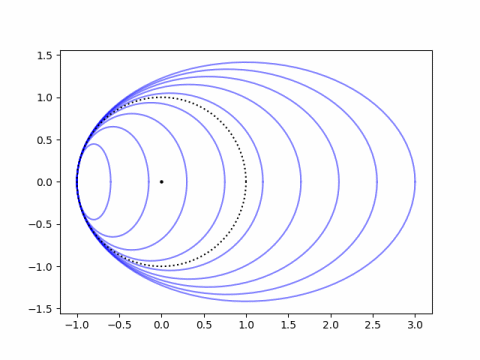An ellipse with equationhas varying curvature at each point. At x = –a the curvature simplifies to a/b². So to make the graph above, I used a range of values for a and set each corresponding value of b to √a.

Next, instead of fixing the osculating circle I’ll fix the curve. I’ll use the equation to fit an egg that I’ve used before and plot the osculating circles at each end. The plot below uses a = 3, b = 2, and k = 0.1.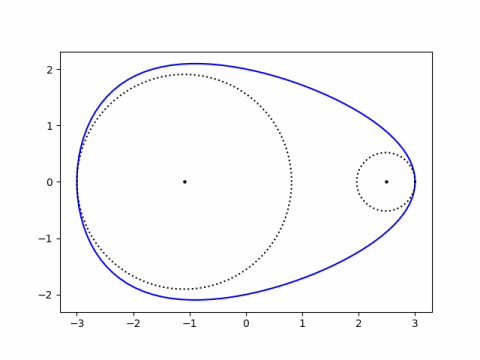The curvature at the fat end is a(1-ka)/b² and the curvature at the pointy end is a(1+ka)/b². These are derived here.

Setting k = 0 gives the curvature of an ellipse at each end used above.

# Surface of revolution with minimum area

Suppose you’re given two points (x1, y1) and (x2, y2) with y1 and y2 positive. Find the smooth positive curve f(x) that passes through the two points such that the area of the surface formed by rotating the graph of f around the x-axis is minimized.

You can state this as a problem in calculus of variations, which leads to a differential equation, which leads to the solution

f(x) = c cosh((x + d)/c).

In other words, the surface area is minimized when the graph of f is a piece of a catenary .

This is interesting because the answer is not something you’re likely to guess, unlike say the isoperimetric problem, where the it’s easy to guess (but hard to prove) that the solution is a circle.

There’s also some interesting fine print to the solution. It’s not quite right to say that the solution is a catenary. To be more precise we should say that if there is a unique catenary that passes through both specified points, then it is the smooth curve with minimal area when rotated about the x-axis. But there are a couple things that could go wrong.

It’s possible that two catenaries pass through the given points, and in that case one of the catenaries leads to minimal surface area. But it’s also possible that there is no catenary passing through the given points.

My first thought would be that you could always find values of c and d so that the function f passes through the points (x1, y1) and (x2, y2), but that’s not true. Often you can, but if the difference in the y‘s is very high relative to the difference in the x‘s it might not be possible.

Suppose the graph of f passes through (0, 1) and (1, y2).

Since the graph passes through the first point, we have

c cosh(d/c) = 1.

Since cosh(x) ≥ 1, we must also have c ≤ 1. And since our curve is positive, we must have c > 0. We can maximize

c cosh((1 + d)/c)

for 0 < c ≤ 1 subject to the constraint

c cosh(d/c) = 1

to find the maximum possible value of y2. If we ask Mathematica

    NMaximize[
{   c Cosh[(1 + d)/c],
{0 < c <= 1},
{c Cosh[d/c] == 1}
},
{c, d}
]


we get

    {6.45659*10^8, {c -> 0.0352609, d -> -0.142316}}

meaning the largest possible value of y2 is 6.45659 × 108, and it occurs when c = 0.0352609, d = -0.142316.

Update: See the comment by Bill Smathers below arguing that the maximum should be unbounded. If the argument is correct, this would imply the code above ran into a numerical limitation.

## Related posts

 See Calculus of Variations by I. M. Gelfand and S. V. Fomin.

# Self-curvature

If you look at a sine wave, its curvature is sorta like its values. When sine is zero, the curve is essentially a straight line, and the curvature is zero. When sine is at its maximum, the function bends the most and so the curvature is at a maximum.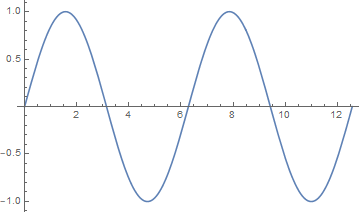This makes sense analytically. The second derivative is something like curvature, and the second derivative of sin(x) is -sin(x). The negative sign suggests that if we look at signed curvature rather than absolute curvature, then the values of a sine curve are roughly proportional to the negative of the curvature at each point.

Here’s a plot showing sin(x) and its negative curvature.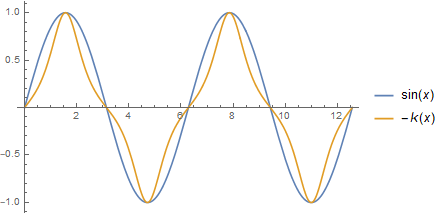Steven Wilkinson wrote an article  addressing the question of whether there is a curve so that the curvature at each point is proportional to the value of the curve. The curvature of a graph of y = y(x) isand so a curve that is proportional to its curvature is a solution to the nonlinear differential equationGeneral theory says that there should at least be a solution in a (possibly small) neighborhood of 0, but it’s not obvious a priori that interesting solutions exist. It turns out that if we pick the proportionality constant a = -1 and the initial conditions y(0) = 0 and y ‘(0) = √3 then we get a periodic solution that looks something like a sine.

The following Mathematica code will plot the function for us.

    s = NDSolve[
{y''[x] = -y[x] (1 + y'[x]^2)^(-3/2),
y == 0, y' == Sqrt},
y, {x, 0, 10}]

Plot[Evaluate[{y[x] /. s}], {x, 0, 10}]


The plot looks a lot like a sine wave. In fact, I imagine that when asked to draw a sine wave, most people would draw something that looks more like this than like an actual sine wave.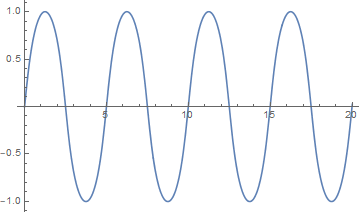## Related posts

 Steven Wilkison. Self-Curvature Curves. Mathematics Magazine, Vol. 82, No. 5 (December 2009), pp. 354-359

# Curvature of an ellipsoid

For an ellipsoid with equationthe Gaussian curvature at each point is given byNow suppose abc > 0. Otherwise relabel the coordinate axes so that this is the case. Then the largest curvature occurs at (±a, 0, 0), and the smallest curvature occurs at (0, 0, ±c).

You could prove this using algebra by manipulating inequalities, or using calculus with Lagrange multipliers.

To see intuitively why this might be true, it helps to exaggerate the shape. First imagine that a is much larger than b or c. Then you have a cigar shape, the greatest curvature as at the two ends. And If you imagine c being much smaller than a and b, you have sort of a pancake shape which is flat on top and bottom.

The maximum curvature is (a/bc)² and the minimum curvature is (c/ab)².

# Total curvature of a knot

Tie a knot in a rope and join the ends together. At each point in the rope, compute the curvature, i.e. how much the rope bends, and integrate this over the length of the rope. The Fary-Milnor theorem says the result must be greater than 4π. This post will illustrate this theorem by computing numerically integrating the curvature of a knot.

If p and q are relatively prime integers, then the following equations parameterize a knot.

x(t) = cos(pt) (cos(qt) + 2)
y(t) = sin(pt) (cos(qt) + 2)
z(t) = -sin(qt)

This is in fact a torus knot because the curve stays on the surface of a torus (doughnut) . We can get a trefoil knot, for example, by setting p = 2 and q = 3.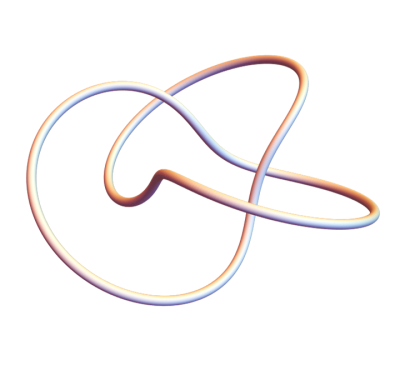We’ll use Mathematica to compute the total curvature of this knot and other examples. First the parameterization:

    x[t_, p_, q_] := Cos[p t] (Cos[q t] + 2)
y[t_, p_, q_] := Sin[p t] (Cos[q t] + 2)
z[t_, p_, q_] := -Sin[q t]


We can plot torus knots as follows.

    k[t_, p_, q_] := {
x[t, p, q],
y[t, p, q],
z[t, p, q]
}
Graphics3D[Tube[Table[k[i, p, q], {i, 0, 2 Pi, 0.01}],
0.08], Boxed -> False]


Here’s a more complicated knot with p = 3 and q = 7.Before we can integrate the curvature with respect to arc length, we need an expression for an element of arc length as a function of the parameter t.

    ds[t_, p_, q_] :=  Sqrt[
D[x[t, p, q], t]^2 +
D[y[t, p, q], t]^2 +
D[z[t, p, q], t]^2
]


Now we can compute the total curvature.

    total[p_, q_] := NIntegrate[
ArcCurvature[k[t, p, q], t] ds[t, p, q],
{t, 0, 2 Pi}
]


We can use this to find that the total curvature of the (2,3) torus knot, the trefoil, is 17.8224, whereas 4π is 12.5664. So the Fary-Milnor theorem holds.

Let’s do one more example, this time a (1,4) knot.You can see that this is not actually knot. This doesn’t contradict what we said above because 1 divides 4. When p or q equal 1, we get an unknot.

When we compute its total curvature we get 24.2737, more than 4π. The Fary-Milnor theorem doesn’t say that total curvature in excess of 4π is a sufficient condition for a loop to be knotted; it says it’s necessary. Total curvature less than 4π proves that something isn’t a knot, but curvature greater than 4π doesn’t prove anything.

## More on curvature and knots

 If you change out the 2s in the parameterization with a larger number, it’s easier to see from the graphs that the curves are on a torus. For example, if we plot the (3,7) knot again, replacing 2’s in the definition of x(t) and y(t) with 5’s, we can kinda see a torus inside the knot.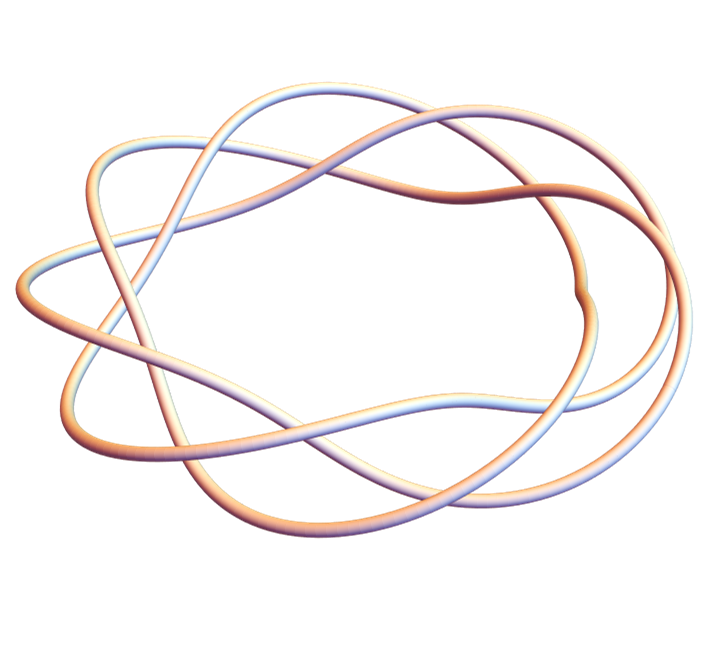# Unit speed curve parameterization

A friend asked me a question the other day that came out of a graphics application. He needed to trace out an ellipse in such a way that the length of curve traced out each second was constant. For a circle, the problem is simple: (cos(t), sin(t)) will trace out a circle covering a constant amount of arc length per unit time. The analogous parameterization for an ellipse, (a cos(t), b sin(t)) will move faster near the longer semi-axis and slower near the shorter one.

There’s a general solution to the problem for any curve. Given a parameterization p(t), where p is vector-valued, the length covered from time 0 to time t isIf you change the time parameterization by inverting this function, solving for t as a function of s, then the total length of curve traversed by p(t(s)) up to time s is s. This is called either the unit speed parameterization or parameterization by arc length.

The hard part is inverting s(t). If you had to find a unit speed parameterization in a calculus class, the problem would be carefully designed so the function s(t) is easy to invert. Real applications don’t usually work out so easily.

## Digression on elliptic integrals and elliptic functions

My response to my friend’s question was that there probably is a closed-form unit speed parameterization, but it would probably involve elliptic functions. He didn’t need much resolution, and decided to do something ad hoc.

Starting with the parameterization  p(t) = (a cos(t), b sin(t)), the arc length s(t) is given by a special function known as an “incomplete elliptic integral of the second kind.” I remembered that the Jacobi elliptic functions were related to the inverses of elliptic integrals, so my hunch was that you could make a unit speed parameterization using Jacobi elliptic functions. Maybe you can, but it’s not as easy as I thought because the Jacobi functions are related to the inverses of elliptic integrals of the first kind.

Elliptic integrals are so named because they were first identified by computing arc length for a (portion of) an ellipse. Elliptic functions were discovered by inverting elliptic integrals, but not the same class of elliptic integrals that give the arc length of an ellipse. (There may well be a transformation that makes these more directly related, but I’m not aware of it.)

Incidentally, elliptic curves are related to elliptic functions, but they are not ellipses! There is a connection from ellipses to elliptic curves, but it’s historical and indirect.

What if we had a more general curve than an ellipse, say something parameterized by cubic splines? Cubic splines are piecewise cubic polynomials, patched together in such a way that the first and second derivatives are continuous across the patches. We can find length of a spline by finding the length of each polynomial patch.

If p(t) is the parameterization of a curve in 2 or 3 dimensions (or really any number of dimensions) and each component of p is a cubic polynomial, then each component of the derivative of p is a quadratic polynomial, and so the sum of the squares of the components is a fourth degree polynomial. So finding the arc length involves integrating the square root of a fourth degree polynomial. This makes it an elliptic integral!

Unfortunately, knowing that the arc length of a cubic spline corresponds to an elliptic integral is not so useful because it could be any type of elliptic integral, depending on its parameters. You’d have to do some work first to put it into a form where you could call on elliptic integrals to finish your problem.

## Numerically computing arc length and unit speed parameterization

The elliptic integral path is something of a dead end. It could still be useful if you needed high accuracy, or if you had some restriction on the class of curves you’re interested in. But in general, you’d need to use numerical integration to find the arc length.

You could also find unit-speed parameterizations numerically, using root-finding to invert s(t) at particular points. Since s is an increasing function of t, you could use a bisection method, which is not the most efficient approach but very robust.

It takes a fair amount of computation to carry root finding where each function evaluation requires computing a numerical integral. But this would work, and depending on your context it could be efficient enough.

If you needed more efficiency, say for a real-time embedded system, you could take a different approach. Your spline is probably an approximation to something else, and so your solution only needs to be as accurate as the spline approximation. This gives you the wiggle room to do something more efficient. You might change your parameterization slightly to make the arc length calculations easier, or come up with a way to approximately invert the arc length function, something that takes advantage of your particular problem and error tolerance.

# Curvature and automatic differentiation

Curvature is tedious to calculate by hand because it involves calculating first and second order derivatives. Of course other applications require derivatives too, but curvature is the example we’ll look at in this post.

## Computing derivatives

It would be nice to write programs that only explicitly implement the original function and let software take care of finding the derivatives.

### Numerical differentiation

Finite difference approximations for derivatives are nothing new. For example, Euler (1707–1783) used finite differences to numerically solve differential equations. But numerical differentiation can be inaccurate or unstable, especially for higher order derivatives.

### Symbolic differentiation

Symbolic differentiation is another approach, having the computer manipulate expressions much as a person would do by hand. It works well for many problems, though it scales poorly for large problems. It also requires functions to be presented in traditional mathematical form, not in the form of source code.

### Automatic differentiation

Automatic differentiation is a third way. Like numerical differentiation, it works with floating point numbers, not symbolic expressions. But unlike numerical differentiation, the result does not have approximation error.

As someone put it, automatic differentiation applies the chain rule to floating point numbers rather than to symbolic expressions.

## Python implementation

I’ll use the Python library autograd to compute curvature and illustrate automatic differentiation. autograd is not the most powerful automatic differentiation library for Python, but it is the simplest I’ve seen.

We will compute the curvature of a logistic curve.The curvature of the graph of a function is given byHere’s Python code using autograd to compute the curvature.

    import autograd.numpy as np

def f(x):
return 1/(1 + np.exp(-x))

f1 = grad(f)  # 1st derivative of f
f2 = grad(f1) # 2nd derivative of f

def curvature(x):
return abs(f2(x))*(1 + f1(x)**2)**-1.5


## Curvature plots

The graph is relatively flat in the middle and at the far ends. In between, the graph bends creating two regions of higher curvature.

    import matplotlib.pyplot as plt

x = np.linspace(-5, 5, 300)
plt.plot(x, f(x))
plt.xlabel("$x$")
plt.ylabel("$y$")
plt.title("Logistic curve")
plt.savefig("logistic_curve.svg")Now let’s look at the curvature.

    y = [curvature(t) for t in x]
plt.plot(x, y)
plt.xlabel("$x$")
plt.ylabel(r"$\kappa(x)$")
plt.title("Curvature")
plt.savefig("plot_logistic_curvature.svg")As we should expect, the curvature is small at the ends and in the middle, with local maxima in between.

We can also look at the signed curvature, the same expression as curvature but without the absolute value.We plot this with the following code.

    def signed_curvature(x):
return f2(x)*(1 + f1(x)**2)**-1.5

y = [signed_curvature(t) for t in x]
plt.plot(x, y)
plt.xlabel("$x$")
plt.ylabel(r"$k(x)$")
plt.title("Signed curvature")
plt.savefig("graph_signed_curvature.svg")


The result looks more like a sine wave.The positive values mean the curve is bending counterclockwise, and the negative values mean the curve is bending clockwise.

Related post: Squircles and curvature